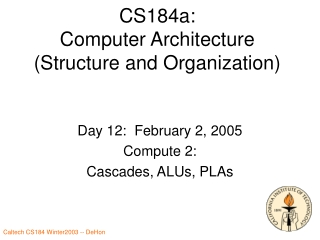# CS184a: Computer Architecture (Structure and Organization) - PowerPoint PPT PresentationDownload PresentationCS184a: Computer Architecture (Structure and Organization)

CS184a: Computer Architecture (Structure and Organization)Download Presentation## CS184a: Computer Architecture (Structure and Organization)

- - - - - - - - - - - - - - - - - - - - - - - - - - - E N D - - - - - - - - - - - - - - - - - - - - - - - - - - -
##### Presentation Transcript

1. CS184a:Computer Architecture(Structure and Organization) Day 12: February 2, 2005 Compute 2: Cascades, ALUs, PLAs

2. Last Time • LUTs • area • structure • big LUTs vs. small LUTs with interconnect • design space • optimization

3. Today • Cascades • ALUs • PLAs

4. Last Time • Larger LUTs • Less interconnect delay • General: Larger compute blocks • Minimize interconnect crossings • Large LUTs • Not efficient for typical logic structure

5. Different Structure • How can we have “larger” compute nodes (less general interconnect) without paying huge area penalty of large LUTs?

6. Small LUTs capture structure What structure does a small-LUT-mapped netlist have? Structure in subgraphs

7. Structure • LUT sequences ubiquitous

8. Hardwired Logic Blocks Single Output

9. Hardwired Logic Blocks Two outputs

10. Delay Model • Tcascade =T(3LUT) + T(mux) • Don’t pay • General interconnect • Full 4-LUT delay

11. Options

12. Chung & Rose Study [Chung & Rose, DAC ’92]

13. Cascade LUT Mappings [Chung & Rose, DAC ’92]

14. ALU vs. Cascaded LUT?

15. Datapath Cascade • ALU/LUT (datapath) Cascade • Long “serial” path w/out general interconnect • Pay only Tmux and nearest-neighbor interconnect

16. 4-LUT Cascade ALU

17. ALU vs. LUT ? • Compare/contrast • ALU • Only subset of ops available • Denser coding for those ops • Smaller • …but interconnect dominates • [Datapath width orthogonal to function]

18. Parallel Prefix LUT Cascade? • Can we do better than N×Tmux? • Can we compute LUT cascade in O(log(N)) time? • Can we compute mux cascade using parallel prefix? • Can we make mux cascade associative?

19. Parallel Prefix Mux cascade • How can mux transform Smux-out? • A=0, B=0  mux-out=0 • A=1, B=1  mux-out=1 • A=0, B=1  mux-out=S • A=1, B=0  mux-out=/S

20. Parallel Prefix Mux cascade • How can mux transform Smux-out? • A=0, B=0  mux-out=0 Stop= S • A=1, B=1  mux-out=1 Generate= G • A=0, B=1  mux-out=S Buffer = B • A=1, B=0  mux-out=/S Invert = I

21. Parallel Prefix Mux cascade • How can 2 muxes transform input? • Can I compute 2-mux transforms from 1 mux transforms?

22. SSS SGG SBS SIG Two-mux transforms • GSS • GGG • GBG • GIS • BSS • BGG • BBB • BII • ISS • IGG • IBI • IIB

23. Generalizing mux-cascade • How can N muxes transform the input? • Is mux transform composition associative?

24. Parallel Prefix Mux-cascade Can be hardwired, no general interconnect

25. “ALU”s Unpacked Traditional/Datapath ALUs • SIMD/Datapath Control • Architecture variable w • Long Cascade • Typically also w, but can shorter/longer • Amenable to parallel prefix implementation in O(log(w)) time w/ O(w) space • Restricted function • Reduces instruction bits • Reduces expressiveness

26. Commercial Devices

27. Xilinx XC4000 CLB

28. Xilinx Virtex-II

29. Altera Stratix

30. Programmable Array Logic(PLAs)

31. PLA • Directly implement flat (two-level) logic • O=a*b*c*d + !a*b*!d + b*!c*d • Exploit substrate properties allow wired-OR

32. Wired-or • Connect series of inputs to wire • Any of the inputs can drive the wire high

33. Wired-or • Implementation with Transistors

34. Programmable Wired-or • Use some memory function to programmable connect (disconnect) wires to OR • Fuse:

35. Programmable Wired-or • Gate-memory model

36. Diagram Wired-or

37. Wired-or array • Build into array • Compute many different or functions from set of inputs

38. Combined or-arrays to PLA • Combine two or (nor) arrays to produce PLA (and-or array) Programmable Logic Array

39. PLA • Can implement each and on single line in first array • Can implement each or on single line in second array

40. PLA • Efficiency questions: • Each and/or is linear in total number of potential inputs (not actual) • How many product terms between arrays?

41. PLA Product Terms • Can be exponential in number of inputs • E.g. n-input xor (parity function) • When flatten to two-level logic, requires exponential product terms • a*!b+!a*b • a*!b*!c+!a*b*!c+!a*!b*c+a*b*c • …and shows up in important functions • Like addition…

42. PLAs • Fast Implementations for large ANDs or ORs • Number of P-terms can be exponential in number of input bits • most complicated functions • not exponential for many functions • Can use arrays of small PLAs • to exploit structure • like we saw arrays of small memories last time

43. PLAs vs. LUTs? • Look at Inputs, Outputs, P-Terms • minimum area (one study, see paper) • K=10, N=12, M=3 • A(PLA 10,12,3) comparable to 4-LUT? • 80-130%? • 300% on ECC (structure LUT can exploit) • Delay? • Claim 40% fewer logic levels (4-LUT) • (general interconnect crossings) [Kouloheris & El Gamal/CICC’92]

44. PLA

45. PLA and Memory